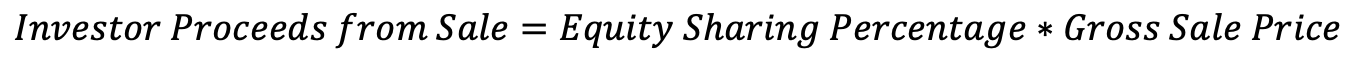Vessel Tutorial
Share
Explore
Tutorial of Math

#Equity Sharing Percentage

Investors are entitled to a portion of the eventual gross sale price of the property. While this portion , the initial equity sharing percentage is simply the investment amount divided by the initial appraised value.
Next topic:
Adjust the sliders below to try it out.
Input:
Investment Amount:
\$
000000
50000
Initial Appraised Home Value:
\$
0000000
500000
Output:
Equity Sharing Percentage:
=
50000
/
500000
=
10
%
Input:
Property sells for:
\$
0000000
600000
Output:
Investor proceeds at time of sale:
=
10
% x \$
600000
= \$
60000
Next topic:
Equity Sharing Percentage
Equity sharing is one of the two main features of home investments through Vessel. When we refer to “buying" or "sharing" equity of a property, what we really mean is buying contractual rights to a percentage of the eventual gross sale price of the property. The initial Equity Sharing Percentage is determined as such:For example, a \$100k investment into a property appraised at \$400k would produce an initial Equity Sharing Percentage of 25%.
Proceeds from Sale
To calculate the amount the investors are owed at the time of sale (or buyout), multiply the equity sharing percentage and the gross sale price of the property. Closing costs are paid in full by the property owner.Notes:
If this amount is greater than the sale price minus the balance due to any mortgage lenders in first position, then this amount would be the balance remaining after all mortgage payments are made.
The Equity Sharing Percentage can increase during the life of the investment through at the property owner's discretions
This is not an actual purchase of equity; however, it uses
legal structure
to simulate something similar.

Share